# Calculus 1 : Calculus

## Example Questions

← Previous 1 3 4 5 6 7 8 9 412 413

### Example Question #1 : How To Find Velocity

If during the first 15 seconds of its flight the displacement of a spacecraft is given by the equation 21.25x + x2 feet, what is its velocity at 10 seconds, given that its initial velocity is 0?

1.25 * 212.5 * log(2) + 20 feet/second

1.25 * 212.5 * log(2) + 100 feet/second

21.25 + 20 feet/second

1.25 * 212.5 + 20 feet/second

1.25 * 212.5 * log(2) + 20 feet/second

Explanation:

To find the velocity function based on displacement, use the first derivative of f(x) = 21.25x + x2.  f'(x) = 1.25 * ln(2) * 21.25x + 2x

The velocity at x = 10 would therefore be: f'(10) = 1.25 * log(2) * 21.25 * 10 + 2 * 10 = 1.25 * 212.5 * log(2) + 20 feet/second

### Example Question #1 : How To Find Velocity

What is the instantaneous velocity at time t = π/2 of a particle whose positional equation is represented by s(t) = 12tan(t/2 + π)?

6

12√2

3√2

6√2

12

12

Explanation:

The instantaneous velocity is represented by the first derivative of the positional equation.

v(t) = s'(t) = 12 * (1/2) sec2(t/2 + π) = 6sec2(t/2 + π) = 6/cos2(t/2 + π) = 6/((–1)2 cos2(t/2)) = 6/cos2(t/2)

Based on the nature of the cosine, we know that 6/cos2(t/2 + π) = 6/((–1)2 cos2(t/2)) = 6/cos2(t/2)

To solve, v(π/2) = 6/cos2(t/2) = 6/((1/√2)2) = 6/(1/2) = 12

### Example Question #1 : Velocity

What is the instantaneous velocity at time t=π/2 of a particle whose positional equation is represented by s(t) = 12cos2(t/2 + π)?

–6

–12

2

2√3

0

–6

Explanation:

The instantaneous velocity is represented by the first derivative of the positional equation.  This is found by using the chain rule both on the square of the cosine function and the function itself.

v(t) = s'(t) = 12 * 2 cos(t/2 + π) * (–sin(t/2 + π)) * (1/2) = –12cos(t/2 + π)sin(t/2 + π)

Given what we know about the cosine and sine functions, we know cos(t/2 + π) = –cos(t/2) and sin(t/2 + π) = –sin(t/2)

Therefore, v(t) = –12(–sin(t/2))(–cos(t/2)) = –12sin(t/2)cos(t/2)

v(π/2) = –12sin(π/4)cos(π/4) = –12(1/√2)(1/√2) = –12 * (1/2) = –6

### Example Question #4 : How To Find Velocity

A weight hanging from a spring is stretched down 3 units beyond its rest position and released at time t=0 to bob up and down. Its position at any later time t is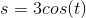What is its velocity at time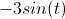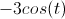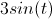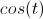Explanation: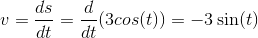### Example Question #1 : How To Find Velocity

The positionof a particle at timeis given by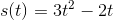.  What is the particle's velocity at time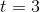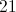Explanation:

The velocity function is given by the derivative of the position function.  So here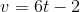.  Plugging 3 in forgives 16.

### Example Question #1 : How To Find Velocity

The position of a particle is given by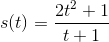.  Find the velocity at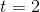.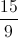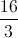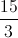Explanation:

The velocity is given as the derivative of the position function, or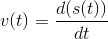.

We can use the quotient rule to find the derivative of the position function and then evaluate that at.  The quotient rule states that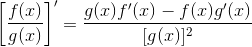.

In this case,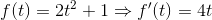and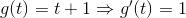.

We can now substitute these values in to get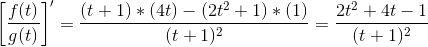.

Evalusting this atgives us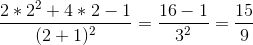.

So the answer is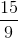.

### Example Question #1 : How To Find Velocity

Find the velocity function if the position function is given as: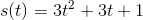.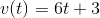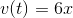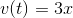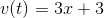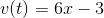Explanation:

There are three terms in this problem that has to be derived.  The derivative of the position function, or the velocity function, represents the slope of the position function.

The derivative of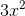can be solved by using the power rule, which is: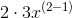Therefore. the derivative ofis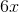.

The derivative ofisby using the constant multiple rule.

The derivative ofissince derivatives of constants equal to zero.### Example Question #1 : How To Find Velocity

Find the velocity function given the position function: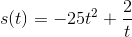.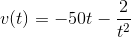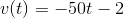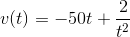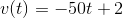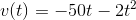Explanation:

The derivatives can be solved term by term.

First, find the derivative of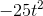.  This can be done by the power rule.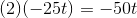Find the derivative of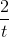.  Rewrite this as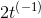to compute by power rule.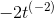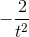Therefore, the velocity function is: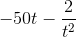### Example Question #1 : How To Find Velocity

Find the velocity of a function if the acceleration is: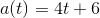.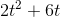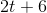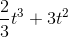Explanation:

To find the velocity given the acceleration function, we will need to integrate the acceleration function.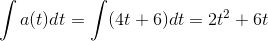### Example Question #1 : How To Find Velocity

Find the velocity atif the acceleration function is: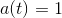.Explanation:

The velocity function can be obtained by integrating the acceleration function.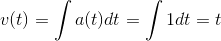Since we are finding the velocity at, substitute this into the velocity function.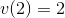← Previous 1 3 4 5 6 7 8 9 412 413

### All Calculus 1 Resources